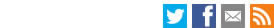Search the LSE website# Abstract:CEP discussion paper
The Log of Gravity
Joao Santos Silva and Silvana Tenreyro
July 2005
Paper No' CEPDP0701:
Full PaperJEL Classification: C13; C21; F10; F11; F12; F15

Although economists have long been aware of Jensen's inequality, many econometric applications have neglected an important implication of it: the standard practice of interpreting the parameters of log-linearized models estimated by ordinary least squares as elasticities can be highly misleading in the presence of heteroskedasticity. This paper explains why this problem arises and proposes an appropriate estimator. Our criticism to conventional practices and the solution we propose extends to a broad range of economic applications where the equation under study is log-linearized. We develop the argument using one particular illustration, the gravity equation for trade, and apply the proposed technique to provide new estimates of this equation. We find significant differences between estimates obtained with the proposed estimator and those obtained with the traditional method. These discrepancies persist even when the gravity equation takes into account multilateral resistance terms or fixed effects